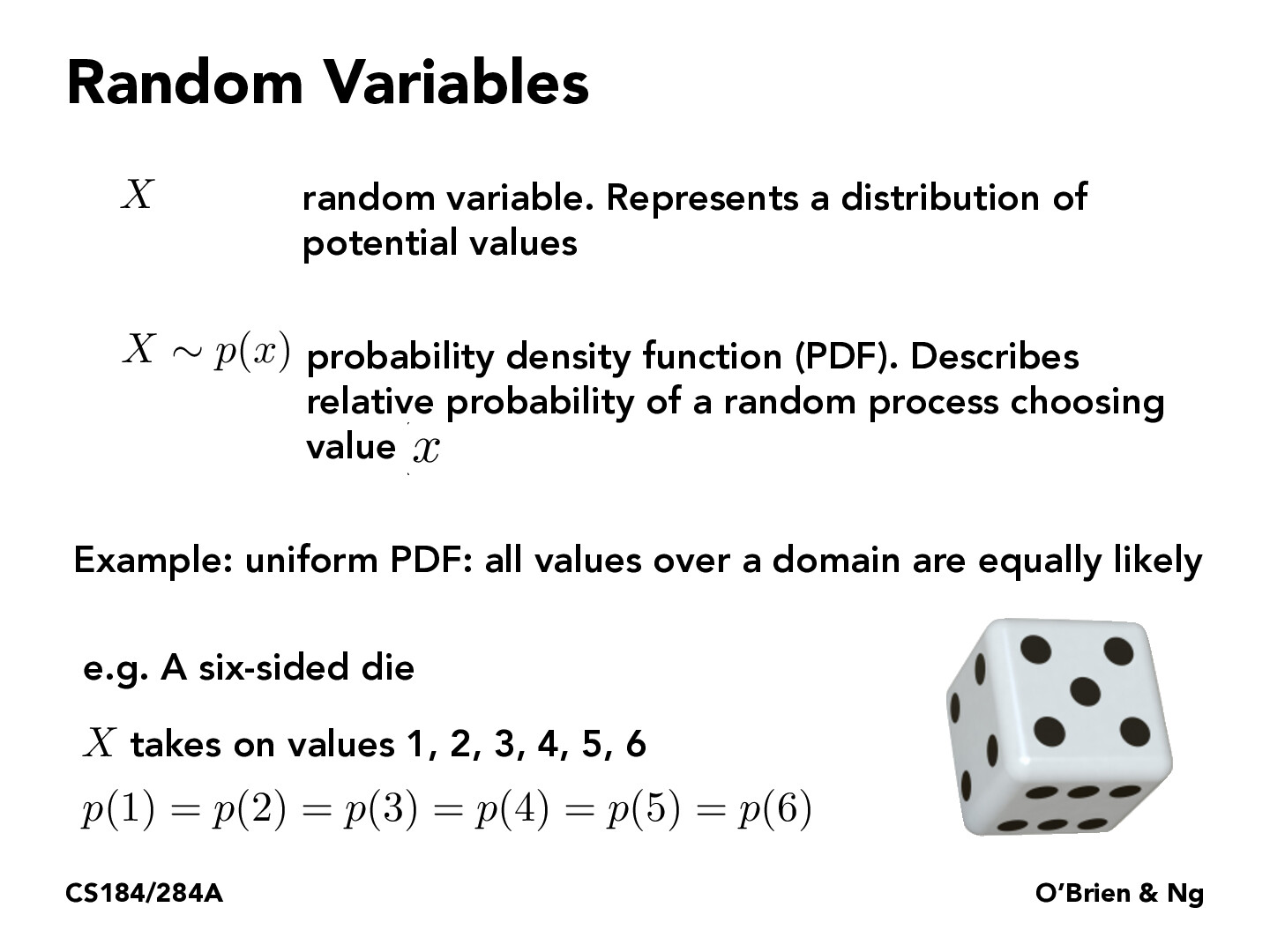Lecture 12: Monte Carlo Integration (14)joeyzhao123

Is the pdf of a uniform dice like a rectangle or a linear line? I'm kind of confused how it's represented.stexus

It would be a rectangle because each each f(x)dx (which sort of represents a probability) would be the same.

You must be enrolled in the course to comment